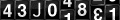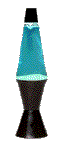# Jack W. Silverstein

### Professor

PhD. Brown University 1975## Jack W. Silverstein on ResearchGateGetting my Institute of Mathematical Statistics
Fellows plaque from Jim Pitman, at the big statistics
meeting in Salt Lake City, July 30, 2007.My fellow ``Fellows''.

### IMS News Release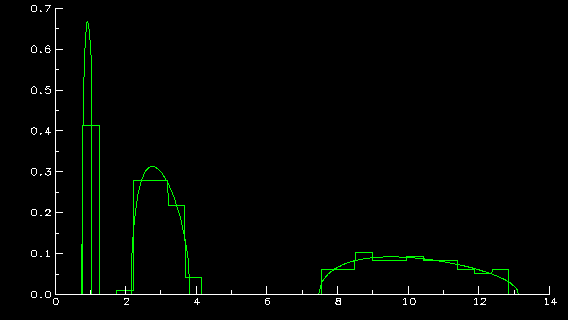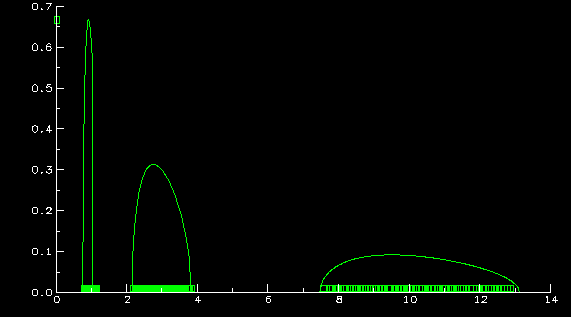Office: 4214 SAS Hall
Phone: (919)515-7864

## Interests

### Spectral properties of large dimensional random matrices

I'll try to explain what the above graphs represent. The histogram in the first one is that of the eigenvalues of a sample covariance matrix (s.c.m.) formed from 4000 samples of a 200 dimensional random vector whose population covariance matrix has 3 distinct eigenvalues: 1, 3, and 10, with respective multiplicities 40, 80, and 80. The assumptions on the random vector are general enough to include multivariate normals. The function you see in both graphs is the limiting density (whose existence and properties are part of my work) of the empirical distribution of the eigenvalues of s.c.m. as the dimension and sample size approach infinity, the population eigenvalues remain at (1,3,10) with relative proportions (.2,.4,.4), and the ratio of dimension to sample size is .05. The closeness of the limiting density to the histogram displays several things: 1) the validity of the above mentioned ``limit theorem'', 2) the ``curse of high dimensionality'', namely that, even though 4000 samples were used to estimate the population matrix, because of the dimension of the random vector, the estimation is not so great, and 3) since the histogram of eigenvalues is predictable from the limit theorem and because of an understanding of how the limiting density should look, due to the relatively small ratio of dimension to sample size (.05), the histogram does reveal quite a bit of information on the eigenvalues of the population matrix (after all, there are 3 clumps centering around the population eigenvalues).

The second graph also contains a scatter plot of all 200 eigenvalues, in clumps of (from left to right) 40, 80, and 80, demonstrating Z.D. Bai and my previous results (see references 32 and 33 in publications): no eigenvalues where they shouldn't be, and the right number where they should be. These two facts, although thoroughly reasonable from the above limit theorem, are a bit more complicated to prove. The limit theorem only guarantees proportion of eigenvalues are converging to the right numbers.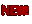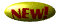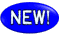# Announcing

## of my latest paper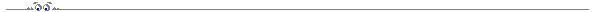# by the Eigenvectors of Large Dimensional Random Matrices## of my latest paper with Yanting Ma, Min Kang, and Dror Baron# Local Convergence of an AMP Variant to the LASSO Solution in Finite Dimensions## of my latest paper with Wlodek Bryc# Singular Values of Large Non-central Random Matrices## of my paper with Katarzyna Bryc and Wlodek Bryc# Separation of the Largest Eigenvalues in Eigenanalysis of Genotype Data from Discrete Subpopulations## of my paper with Romain Couillet and Frédéric Pascal# Robust M-Estimation for Array Processing: A Random Matrix Approach## of my paper with Walid Hachem, Malika Kharouf, and Jamal Najim# A CLT for Information-theoretic Statistics of Non-centered Gram Random Matrices## of my paper with Zhidong Bai# No Eigenvalues Outside the Support of the Limiting Spectral Distribution of Information-Plus Noise Type Matrices## of my paper with Romain Couillet, Zhidong Bai, and Merouane Debbah# Eigen-inference for Energy Estimation of Multiple Sources## and## the book Zhidong Bai and I have written:# Spectral Analysis of Large Dimensional Random Matrices

## Springer Series in Statistics, 2009###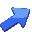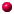MA 544: Computer Experiments in Mathematical Probability

My favorite groupThe best children's book seriesWrite to me at jack at math ncsu edu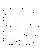Department of Mathematics, Box 8205
North Carolina State University
Raleigh, NC 27695-8205(December, 2020)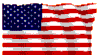You are visitor number: